Open In App

# Python | Pandas Series.pop()

Pandas series is a One-dimensional ndarray with axis labels. The labels need not be unique but must be a hashable type. The object supports both integer- and label-based indexing and provides a host of methods for performing operations involving the index.

Pandas` Series.pop()` function returns the item corresponding to the passed index label. It also drops the item from the series object.

Syntax: Series.pop(item)

Parameter :
item : str

Returns : popped : Series

Example #1: Use `Series.pop()` function to pop an element from the given series object.

 `# importing pandas as pd``import` `pandas as pd`` ` `# Creating the Series``sr ``=` `pd.Series([``10``, ``25``, ``3``, ``11``, ``24``, ``6``])`` ` `# Create the Index``index_ ``=` `[``'Coca Cola'``, ``'Sprite'``, ``'Coke'``, ``'Fanta'``, ``'Dew'``, ``'ThumbsUp'``]`` ` `# set the index``sr.index ``=` `index_`` ` `# Print the series``print``(sr)`

Output :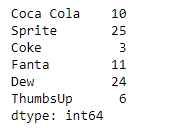Now we will use `Series.pop()` function to pop the entry for ‘Sprite’ in the given Series object.

 `# pop the passed index label from``# series object``result ``=` `sr.pop(item ``=` `'Sprite'``)`` ` `# Print the result``print``(result)`` ` `# Series after popping ``print``(sr)`

Output :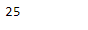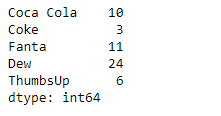As we can see in the output, the `Series.pop()` function has successfully popped the entry for the passed label in the given series object.

Example #2 : Use `Series.pop()` function to pop an element from the given series object. The given series object contains some missing values in it.

 `# importing pandas as pd``import` `pandas as pd`` ` `# Creating the Series``sr ``=` `pd.Series([``19.5``, ``16.8``, ``None``, ``22.78``, ``None``, ``20.124``, ``None``, ``18.1002``, ``None``])`` ` `# Print the series``print``(sr)`

Output :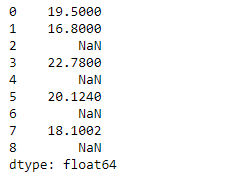Now we will use `Series.pop()` function to pop the entry corresponding to the 1st index label in the given Series object.

 `# pop the passed index label from``# series object``result ``=` `sr.pop(item ``=` `1``)`` ` `# Print the result``print``(result)`` ` `# Series after popping ``print``(sr)`

Output :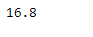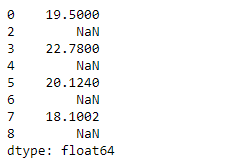As we can see in the output, the `Series.pop()` function has successfully popped the entry for the passed label in the given series object.